# 图像形态学提取边界和区域填充

2019/04/10 10:10

1、二值图像边界提取、轮廓提取

``````imgPath = 'E:\opencv_pic\src_pic\pic1.bmp';
img=rgb2gray(img);
img = 255-img;

se = strel('square', 5);
img2 = imerode(img, se);
subplot(1,3,1),imshow(255-img),title('原始图像');
subplot(1,3,2),imshow(255-img2),title('腐蚀图像');
subplot(1,3,3),imshow(255-(img-img2)),title('边界点');``````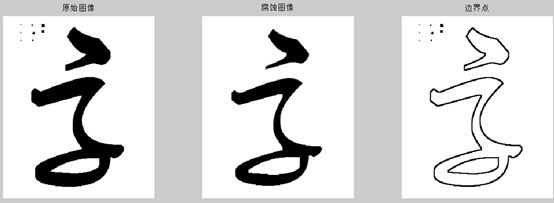2、形态学方法图像区域填充

a）原始图像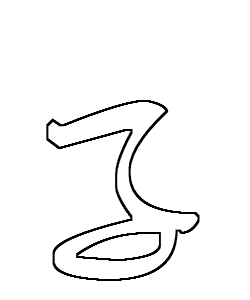b）读取图像A以及补集Ac

``````imgPath = 'E:\opencv_pic\src_pic\pic1-1.bmp';
img0 = 255-img1; % 取反景图
subplot(1,2,1),imshow(img1),title('原始图像');
subplot(1,2,2),imshow(img0),title('补集图像');``````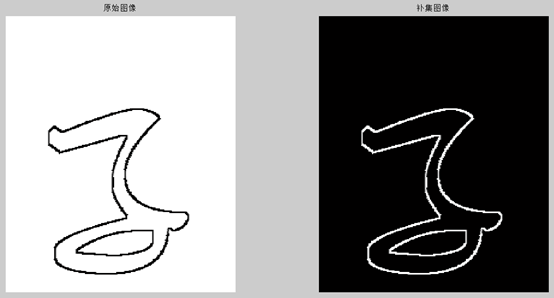c）图像膨胀

``````figure;
[m,n]= size(img0);
B0 = zeros(m,n);
B0(50, 150) = 1;
se = [0 1 0;1 1 1;0 1 0];
B1=imdilate(B0, se) & img1;
B2=imdilate(B1, se) & img1;
subplot(1,3,1),imshow(B0),title('结构元素se');
subplot(1,3,2),imshow(B1),title('腐蚀B1');
subplot(1,3,3),imshow(B2),title('腐蚀B2');``````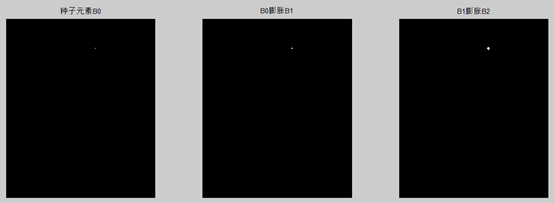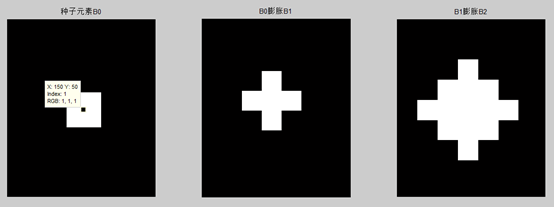d）循环膨胀、填充区域增长

``````figure;
while 1
imshow(B2);
if B1 == B2
break;
else
B1 = B2;
B2=imdilate(B1,se) & img1;
end
end``````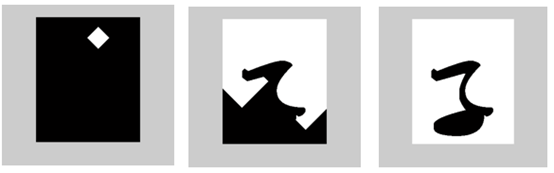填充过程如下图，先从B0开始膨胀，循环膨胀图像不断生长，当图像超出边界后与背景图像（补集）相交限制图像的边界。直到图像无法再生长，图像完成内部区域填充。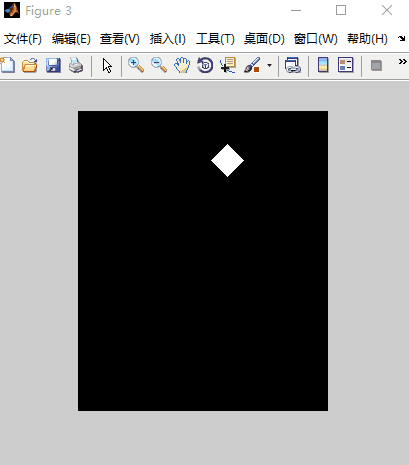上面的动图选的起点种子元素在图像区域外部，白色区域，所以填充过程白色区域膨胀然后只保留了图像的内部区域为黑色。如果起始点种子元素在区域内部，则白色块膨胀被图像的边界限制无法突破边界，最终白色点越来越多，直至完成区域填充。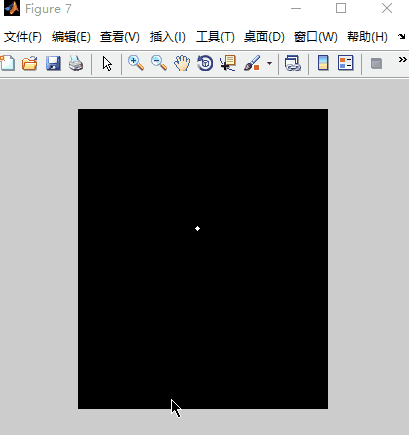3、参考文献

1、《数字图像处理与机器视觉》，张铮、徐超、任淑霞、韩海玲编著

0
0 收藏

### 作者的其它热门文章0 评论
0 收藏
0